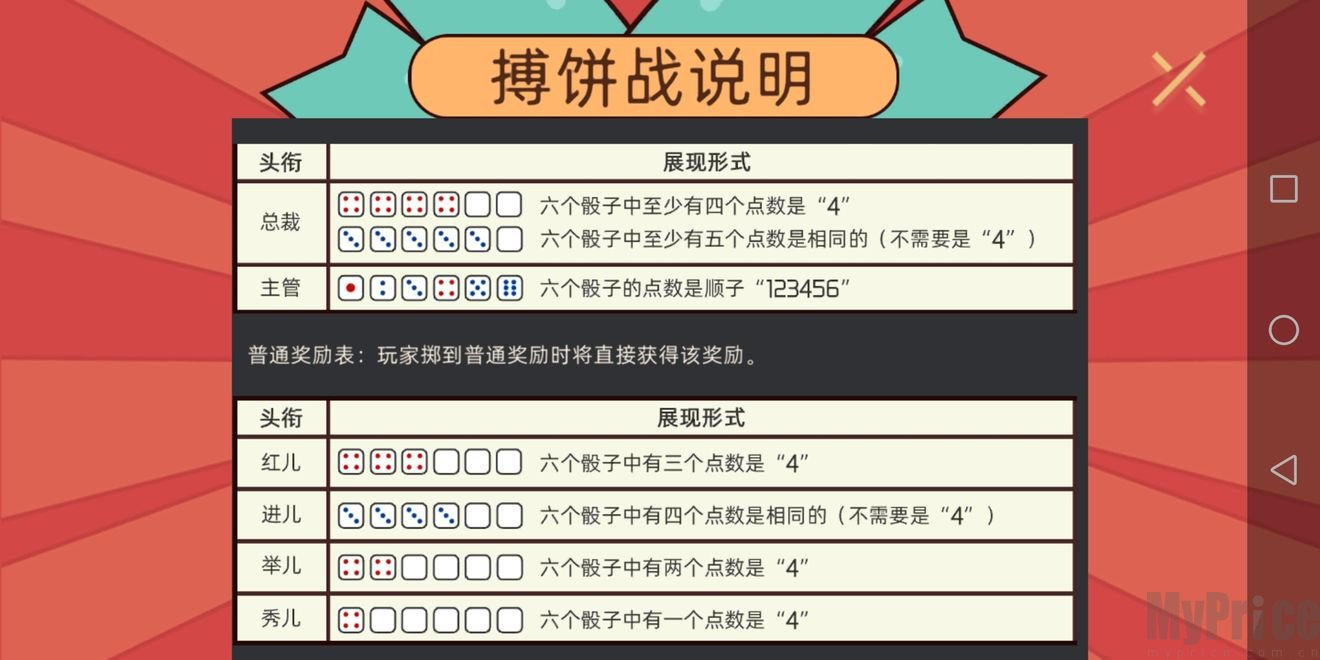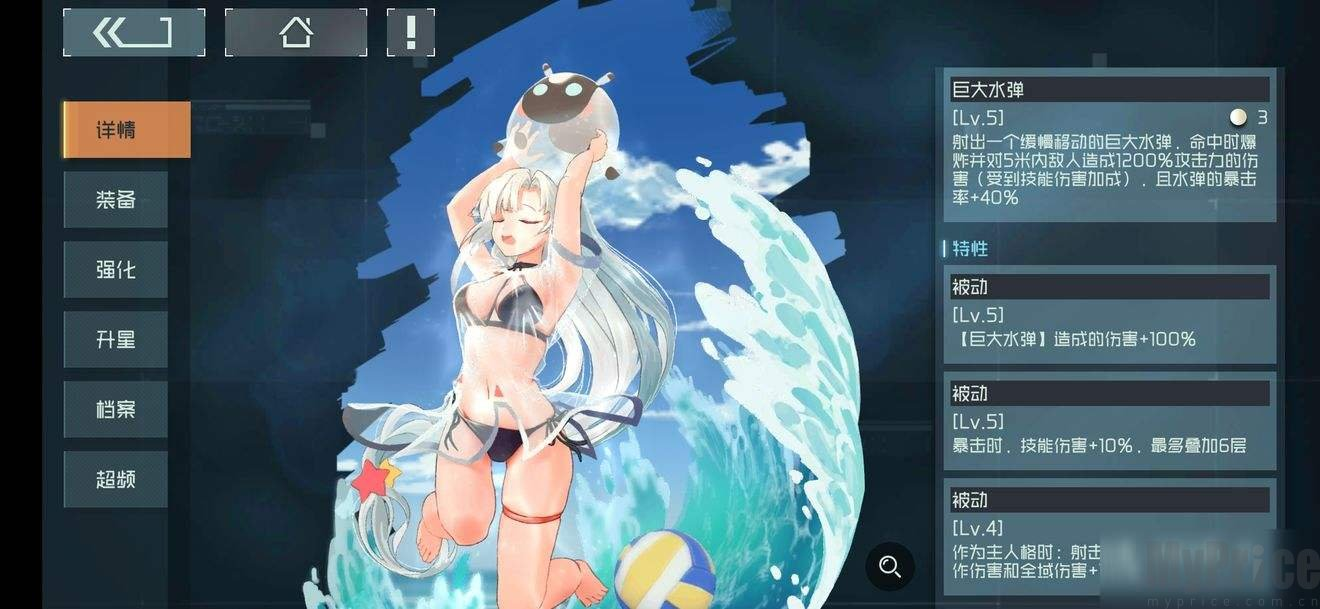### 映月城与电子姬搏饼战概率详情P(总裁)

= P(只有四个4) + P(只有五个X) + P(六个X)

= [C(6,4) * 5^2 + 6 * C(6,5) * 5 + 6] / 6^6

= 561 / 46656

= 1.202%

P(主管)

= P(顺子)

= 6! / 6^6

= 720 / 46656

= 1.543%

P(红儿)

= P(只有三个4)

= C(6,3) * 5^3 / 6^6

= 2500 / 46656

= 5.358%

P(进儿)

= P(只有四个X，X不取4)

= 5 * C(6,4) * 5^2 / 6^6

= 1875 / 46656

= 4.019%

P(举儿)

= P(只有两个4)

= C(6,2) * 5^4 / 6^6

= 9375 / 46656

= 20.094%

P(秀儿)

= P(只有一个4) - P(顺子)

= [C(6,1) * 5^5 - 6!] / 6^6

= 18030 / 46656

= 38.635%

P(啥都没有)

= 29.139%### 游戏推荐